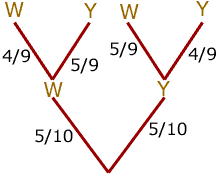Quandaries and Queries Question: Having trouble with this one. There are 5 white and 5 yellow balls in a golf bag. Two are selected randomly, and simultaneously. What is the probability that the first one or the second one will be white? Hi, I would draw a tree diagram. On my diagram W is selecting a white ball and Y is selecting a yellow ball.There are 4 ways to progress through the tree and 3 of them have at least one white ball being selected. The only path through the tree with no white ball selected is to obtain a yellow ball on the first selection and a yellow ball on the second selection. From the tree diagram the first has probability 5/10 and the second has probability 4/9. Thus the probability that you select no white balls is 5/104/9 = 2/9 Hence the probability that either the first ball is white or the second ball is white is 1 - 2/9 = 7/9 Penny Go to Math Central